## Tuesday, August 7, 2007

### Irodov Problem 1.67The figure beside depicts the force diagram. Let us try and find the tension T in the string in the problem. Let the mass be m, and further suppose that the mass is being accelerated along the upwards slope at an rate w. Further let us resolve the forces along the directions parallel and perpendicular to the direction of the inclined plane.

Along the perpendicular direction, there are three forces - i) the normal reaction from the surface of the inclined plane N, ii) the component of gravity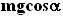and iii) the component of the tension in the string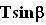. There is no component of acceleration for the mass along the perpendicular direction. Thus, we have,Along the direction parallel to the incline, we have 3 forces acting on the mass - i) the component of gravitytrying to pull the mass down the inclined plane, ii) the component of tension pulling the mass upwardsand iii) the force of friction that opposes the motion of the mass acting downwards (to oppose the upward motion) Nk. We have assumed that the mass accelerates at a rate w and so we have,Thus, the minimum in tension T can be determined by maximizing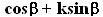. We can find the extrema (maxima or minima) ofby differentiating it with respect to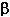and equating this to 0. Thus we have,Now for this value ofto be the maxima its second derivative must be negative. The second derivative is given by,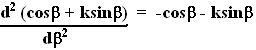which is negative throughout 0 to 90 degrees. Thus, the above obtained value ofcorresponds to the minimum tension in the string.

The actual value of this minimum tension in the string can determined by using this obtained value ofin (3) as,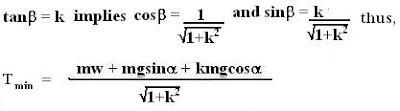Further, the value of tension will be minimized at w=0 (the mass is pulled up at constant speed) , thus we obtain,